# Machine Learning Lecture 9 Rule Learning Inductive Logic

• Slides: 22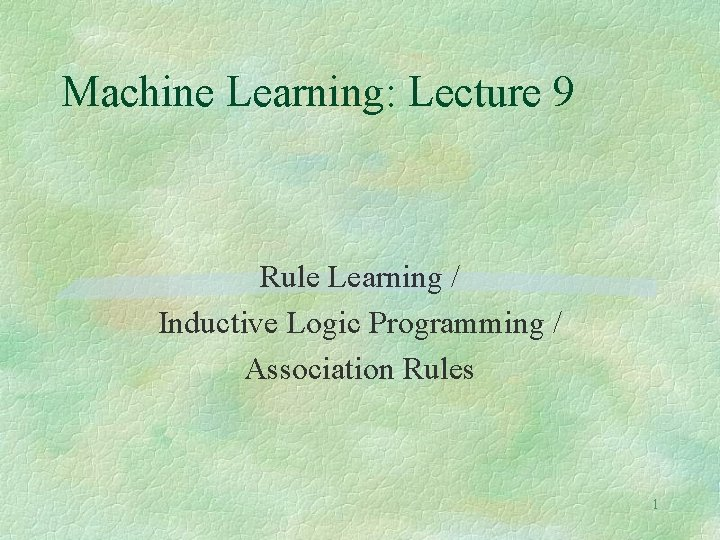Machine Learning: Lecture 9 Rule Learning / Inductive Logic Programming / Association Rules 1Learning Rules § § § One of the most expressive and human readable representations for learned hypotheses is sets of production rules (if-then rules). Rules can be derived from other representations (e. g. , decision trees) or they can be learned directly. Here, we are concentrating on the direct method. An important aspect of direct rule-learning algorithms is that they can learn sets of first-order rules which have much more representational power than the propositional rules that can be derived from decision trees. Rule Learnng also allows the incorporation of background knowledge nto the process. Learning rules is also useful for the data mining task of association rules mining. 2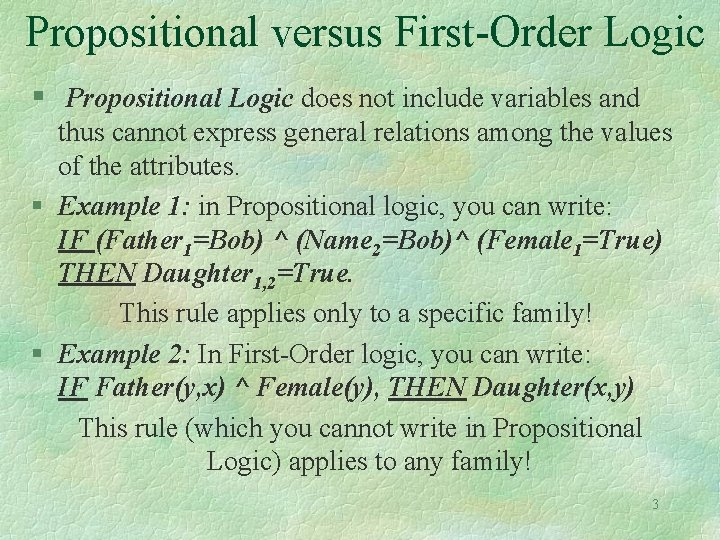Propositional versus First-Order Logic § Propositional Logic does not include variables and thus cannot express general relations among the values of the attributes. § Example 1: in Propositional logic, you can write: IF (Father 1=Bob) ^ (Name 2=Bob)^ (Female 1=True) THEN Daughter 1, 2=True. This rule applies only to a specific family! § Example 2: In First-Order logic, you can write: IF Father(y, x) ^ Female(y), THEN Daughter(x, y) This rule (which you cannot write in Propositional Logic) applies to any family! 3Learning Propositional versus First-Order Rules § Both approaches to learning are useful as they address different types of learning problems. § Like Decision Trees, Feedforward Neural Nets and IBL systems, Propositional Rule Learning systems are suited for problems in which no substantial relationship between the values of the different attributes needs to be represented. § In First-Order Learning Problems, the hypotheses that must be represented involve relational assertions that can be conveniently expressed using first-order representations such as horn clauses (H <- L 1 ^…^Ln). 4Learning Propositional Rules: Sequential Covering Algorithms Sequential-Covering(Target_attribute, Attributes, Examples, Threshold) § Learned_rules <-- { } § Rule <-- Learn-one-rule(Target_attribute, Attributes, Examples) § While Performance(Rule, Examples) > Threshold, do l Learned_rules <-- Learned_rules + Rule l Examples <-- Examples -{examples correctly classified by Rule} l Rule <-- Learn-one-rule(Target_attribute, Attributes, Examples) § Learned_rules <-- sort Learned_rules according to Performance over Examples § Return Learned_rules 5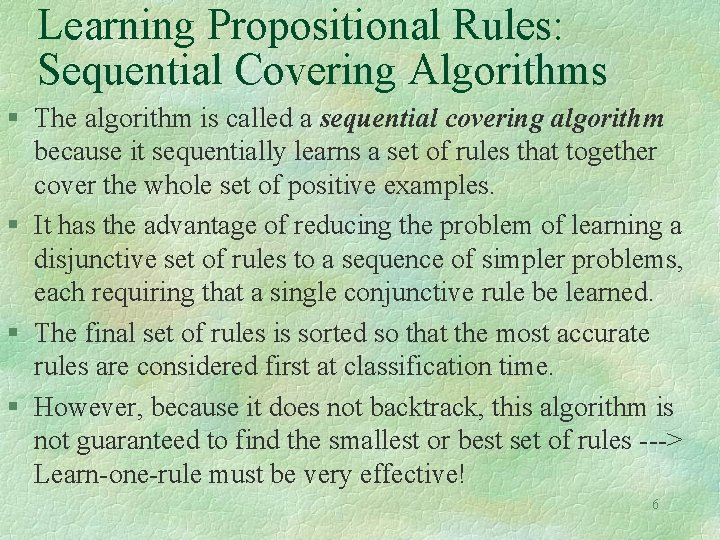Learning Propositional Rules: Sequential Covering Algorithms § The algorithm is called a sequential covering algorithm because it sequentially learns a set of rules that together cover the whole set of positive examples. § It has the advantage of reducing the problem of learning a disjunctive set of rules to a sequence of simpler problems, each requiring that a single conjunctive rule be learned. § The final set of rules is sorted so that the most accurate rules are considered first at classification time. § However, because it does not backtrack, this algorithm is not guaranteed to find the smallest or best set of rules ---> Learn-one-rule must be very effective! 6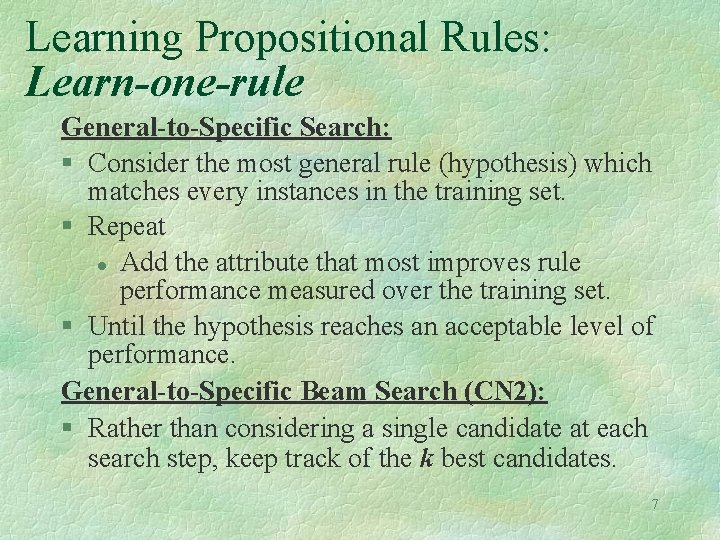Learning Propositional Rules: Learn-one-rule General-to-Specific Search: § Consider the most general rule (hypothesis) which matches every instances in the training set. § Repeat l Add the attribute that most improves rule performance measured over the training set. § Until the hypothesis reaches an acceptable level of performance. General-to-Specific Beam Search (CN 2): § Rather than considering a single candidate at each search step, keep track of the k best candidates. 7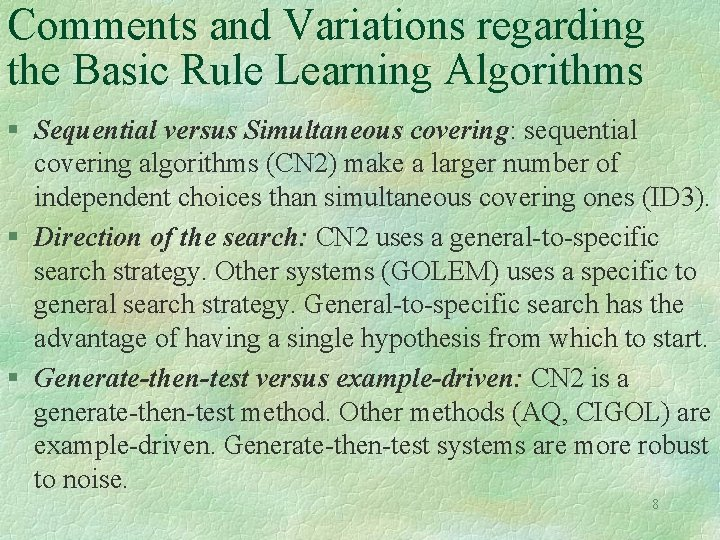Comments and Variations regarding the Basic Rule Learning Algorithms § Sequential versus Simultaneous covering: sequential covering algorithms (CN 2) make a larger number of independent choices than simultaneous covering ones (ID 3). § Direction of the search: CN 2 uses a general-to-specific search strategy. Other systems (GOLEM) uses a specific to general search strategy. General-to-specific search has the advantage of having a single hypothesis from which to start. § Generate-then-test versus example-driven: CN 2 is a generate-then-test method. Other methods (AQ, CIGOL) are example-driven. Generate-then-test systems are more robust to noise. 8Comments and Variations regarding the Basic Rule Learning Algorithms, Cont’d § Post-Pruning: pre-conditions can be removed from the rule whenever this leads to improved performance over a set of pruning examples distinct from the training set. § Performance measure: different evaluation can be used. Example: relative frequency (AQ), mestimate of accuracy (certain versions of CN 2) and entropy (original CN 2). 9Example: RIPPER (this and the next three slides are borrowed from E. Alpaydin, Lecture Notes for An Introduction to machine Learning, 2004, MIT Press. (Chapter 9)). There are two kinds of loop in the Ripper algorithm: Outer loop : adding one rule at a time to the rule base Inner loop : adding one condition at a time to the current rule Conditions are added to the rule to maximize an information gain measure. Conditions are added to the rule until it covers no negative example. 10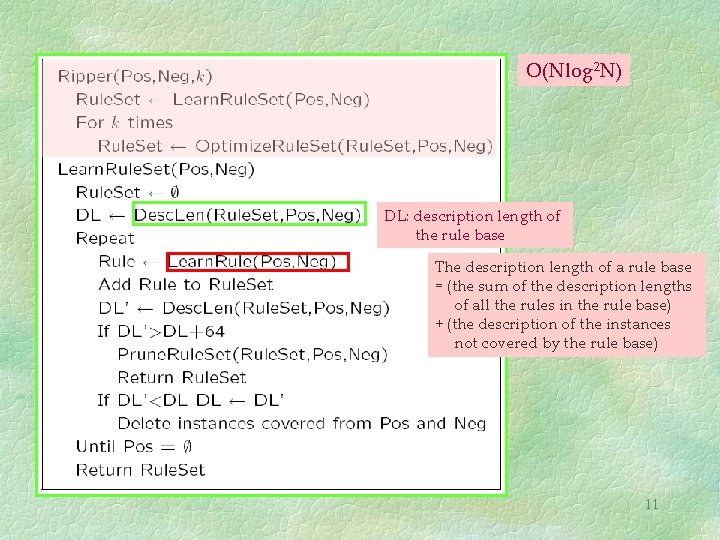O(Nlog 2 N) DL: description length of the rule base The description length of a rule base = (the sum of the description lengths of all the rules in the rule base) + (the description of the instances not covered by the rule base) 1112Ripper Algorithm § In Ripper, conditions are added to the rule to l Maximize an information gain measure • • • l R : the original rule R’ : the candidate rule after adding a condition N (N’): the number of instances that are covered by R (R’) N+ (N’+): the number of true positives in R (R’) s : the number of true positives in R and R’ (after adding the condition) Until it covers no negative example p and n : the number of true and false Rule value metric positives respectively. 13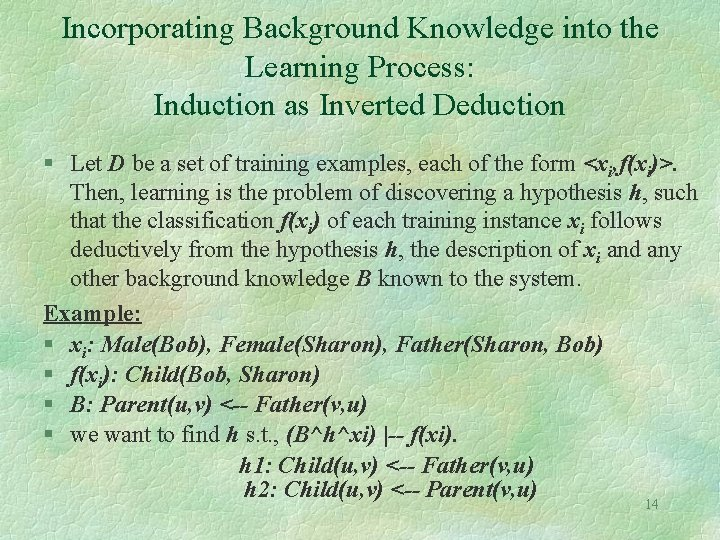Incorporating Background Knowledge into the Learning Process: Induction as Inverted Deduction § Let D be a set of training examples, each of the form <xi, f(xi)>. Then, learning is the problem of discovering a hypothesis h, such that the classification f(xi) of each training instance xi follows deductively from the hypothesis h, the description of xi and any other background knowledge B known to the system. Example: § xi: Male(Bob), Female(Sharon), Father(Sharon, Bob) § f(xi): Child(Bob, Sharon) § B: Parent(u, v) <-- Father(v, u) § we want to find h s. t. , (B^h^xi) |-- f(xi). h 1: Child(u, v) <-- Father(v, u) h 2: Child(u, v) <-- Parent(v, u) 14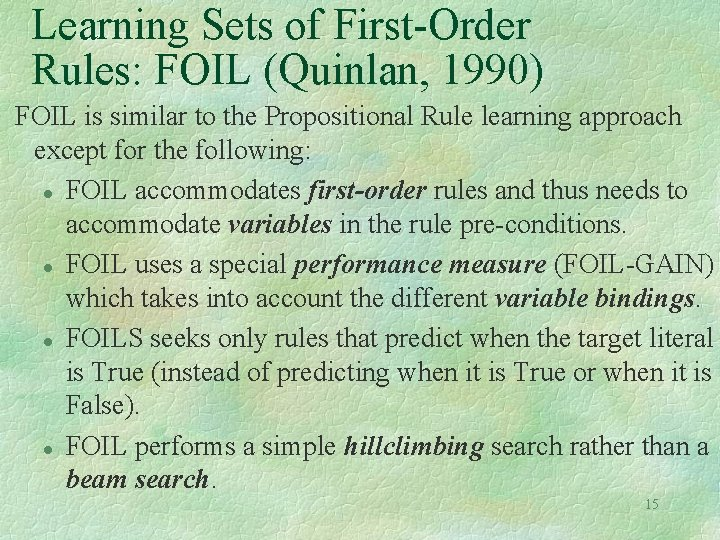Learning Sets of First-Order Rules: FOIL (Quinlan, 1990) FOIL is similar to the Propositional Rule learning approach except for the following: l FOIL accommodates first-order rules and thus needs to accommodate variables in the rule pre-conditions. l FOIL uses a special performance measure (FOIL-GAIN) which takes into account the different variable bindings. l FOILS seeks only rules that predict when the target literal is True (instead of predicting when it is True or when it is False). l FOIL performs a simple hillclimbing search rather than a beam search. 15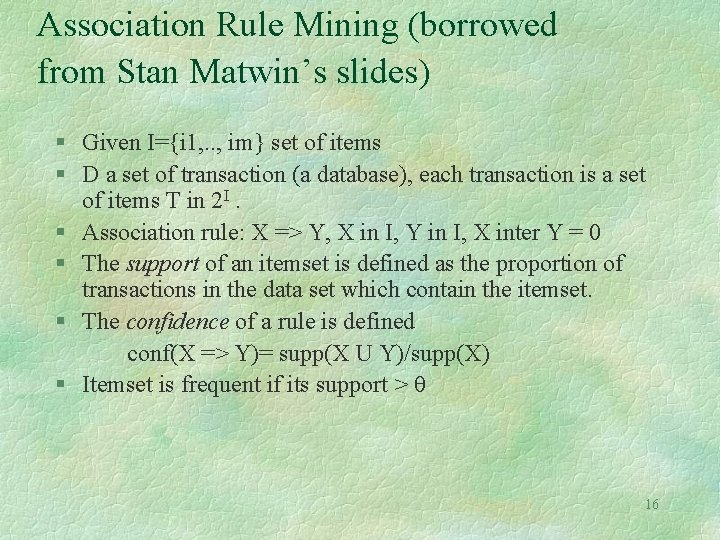Association Rule Mining (borrowed from Stan Matwin’s slides) § Given I={i 1, . . , im} set of items § D a set of transaction (a database), each transaction is a set of items T in 2 I. § Association rule: X => Y, X in I, Y in I, X inter Y = 0 § The support of an itemset is defined as the proportion of transactions in the data set which contain the itemset. § The confidence of a rule is defined conf(X => Y)= supp(X U Y)/supp(X) § Itemset is frequent if its support > θ 16Itemsets and Association Rules § Itemset = set of items § k-itemset = set of k-items § Finding association rules in databases: l l Find all frequent (or large) itemsets (those with support > min. S) Generate rules that satisfy minimum confidence § Example of an association rule: People who buy a computer also buy financial software (support of 2%; confidence of 60%) 17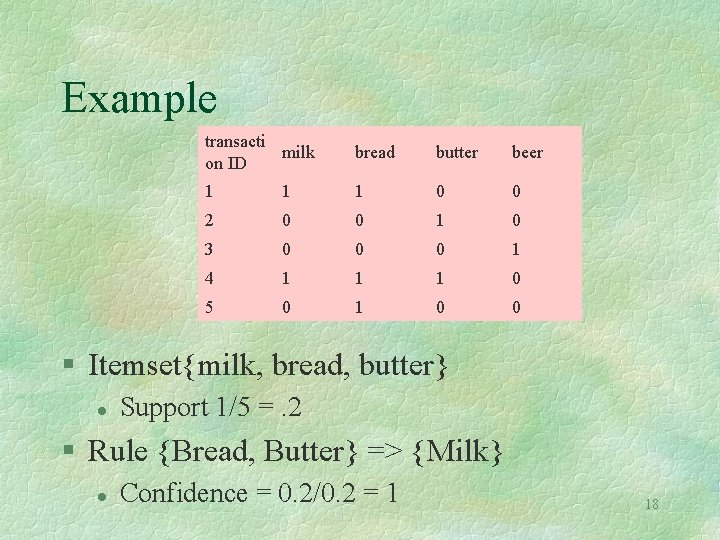Example transacti milk on ID bread butter beer 1 1 1 0 0 2 0 0 1 0 3 0 0 0 1 4 1 1 1 0 5 0 1 0 0 § Itemset{milk, bread, butter} l Support 1/5 =. 2 § Rule {Bread, Butter} => {Milk} l Confidence = 0. 2/0. 2 = 1 18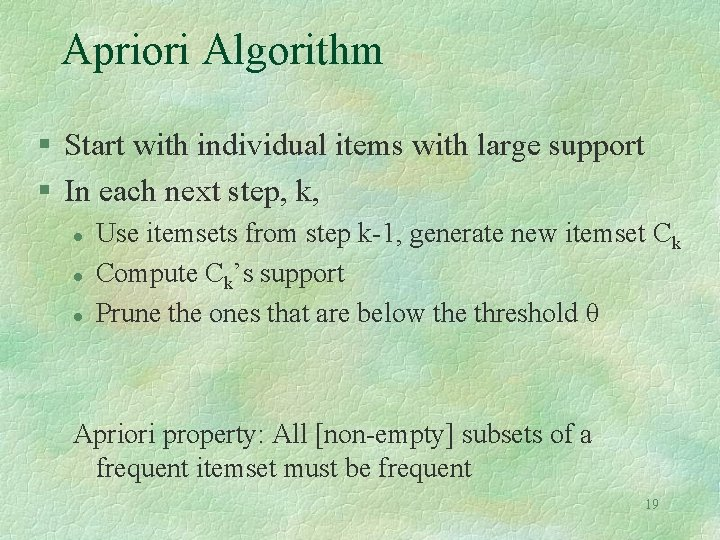Apriori Algorithm § Start with individual items with large support § In each next step, k, l l l Use itemsets from step k-1, generate new itemset Ck Compute Ck’s support Prune the ones that are below the threshold θ Apriori property: All [non-empty] subsets of a frequent itemset must be frequent 19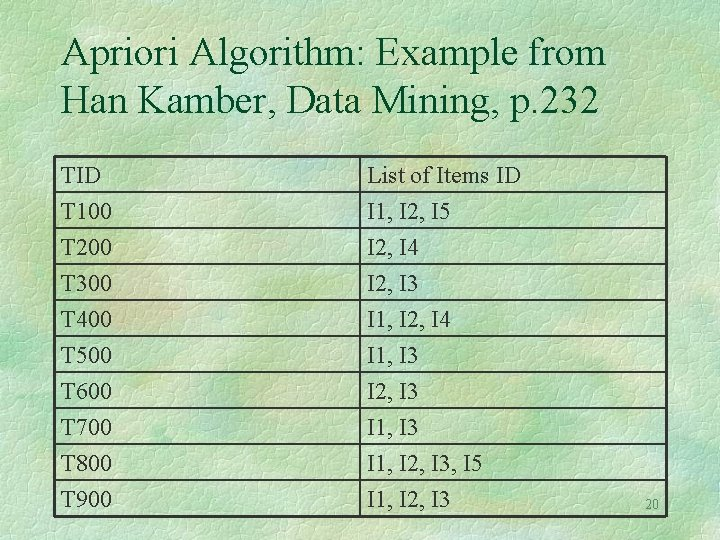Apriori Algorithm: Example from Han Kamber, Data Mining, p. 232 TID T 100 T 200 T 300 List of Items ID I 1, I 2, I 5 I 2, I 4 I 2, I 3 T 400 T 500 T 600 T 700 T 800 T 900 I 1, I 2, I 4 I 1, I 3 I 2, I 3 I 1, I 2, I 3, I 5 I 1, I 2, I 3 20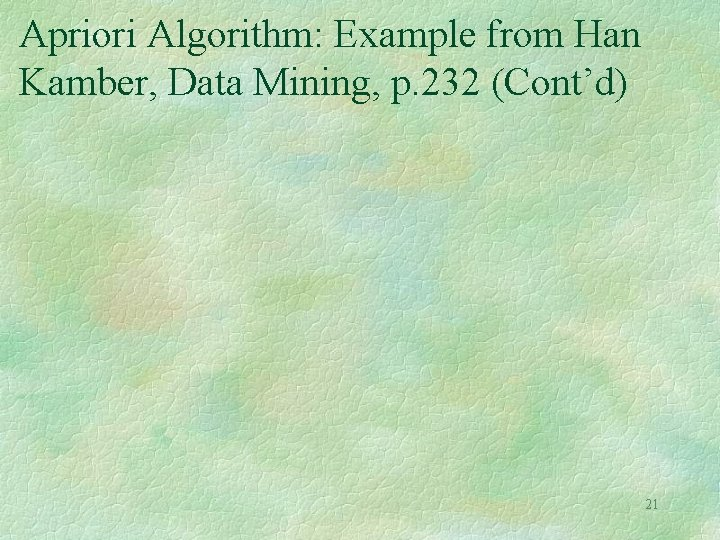Apriori Algorithm: Example from Han Kamber, Data Mining, p. 232 (Cont’d) 21From itemsets to association rules § For each Frequent itemset I l generate all the partitions of I into s, I-s § Attempt a rule s => I-s iff support_count(I)/support_count(s) > minc 22